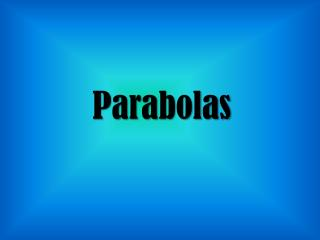DownloadDownload PresentationParabolas

# Parabolas

Télécharger la présentation## Parabolas

- - - - - - - - - - - - - - - - - - - - - - - - - - - E N D - - - - - - - - - - - - - - - - - - - - - - - - - - -
##### Presentation Transcript

1. Parabolas

2. We already know A LOT about parabolas • 2 forms (standard and vertex) • How to find Vertex (h,k) or (-b/2a) • Axis of Symmetry • Characteristics • Many ways to solve their equations • Solutions are x intercepts

3. We are going to add a couple things • Focus and Directrix • Parabolas that are turned to left and right

4. Focus and Directrix • The Focus is a point inside the parabola • The directrix is a line outside the parabola • All points on the parabola are equidistant from the focus and directrix • The vertex is midway between the focus and directrix

5. Here are some other applications of the focus...

6. The distance from the vertex to the focus (or the vertex to the directrix) is called p

7. Vertex Form Equation of a Parabola Horizontal Parabola Vertical Parabola Vertex: (h, k) Vertex: (h, k) If 4p > 0, opens right If 4p > 0, opens up If 4p < 0, opens left If 4p < 0, opens down The directrix is vertical (x= ) The directrix is horizontal (y= ) Remember: |p| is the distance from the vertex to the focus

8. Find the focus and equation of the directrix. Then sketch the graph. Opens up

9. Find the focus and equation of the directrix. Then sketch the graph. Opens right Vertex (0,0)

10. Example: x = -1/16 (y – 2)2+ 5 : Direction: Vertex: Focus: Directrix:

11. Example: Determine the focus and directrix of the parabola y = 1/8 (x – 8)2- 3 : Direction: Vertex: Focus: Directrix:

12. Converting an Equation Convert the equation to standard form Find the vertex, focus, and directrix y2 – 2y + 12x – 35 = 0 1 y2 – 2y + ___ = -12x + 35 + ___ 1 (y – 1)2 = -12x + 36 F (y – 1)2 = -12(x – 3) V x = -1/12 (y – 1)2+ 3 The parabola is horizontal and opens left Vertex: (3, 1) 4p = -12 Focus: (0, 1) p = -3 Directrix: x = 6

13. Write the equation in standard form by completing the square. State the VERTEX, focus, and directrix.

14. Write the equation in standard form by completing the square. State the VERTEX, focus, and directrix.

15. Write the equation of a parabola with vertex (-4, -1) that has a focus (-4, 2) Find p 3

16. Write the equation of a parabola with vertex (1, 2) that has a focus (5, 2) 4 Find p

17. Find the standard form of the equation of the parabola given: the focus is (2, 4) and the directrix is x = - 4 The vertex is midway between the focus and directrix, so the vertex is (-1, 4) The directrix is vertical so the parabola must be horizontal and since the focus is always inside the parabola, it must open to the right V F Equation: x=1/4p (y – k)2+ h |p| = 3 Equation:x= 1/12 (y – 4)2- 1

18. A parabola has its focus at (1, -2) and its directrix at y = 2. Does the point (5, -2) lie on the parabola?

19. Applications A satellite dish is in the shape of a parabolic surface. The dish is 12 ft in diameter and 2 ft deep. How far from the base should the receiver be placed? 12 2 (-6, 2) (6, 2) Consider a parabola cross-section of the dish and create a coordinate system where the origin is at the base of the dish. Since the parabola is vertical and has its vertex at (0, 0) its equation must be of the form: y = 1/4p x2 The receiver should be placed 4.5 feet above the base of the dish. At (6, 2), 2 = 1/4p (36) so p = 4.5